# Four WHL Clubs in the Mosaik MasterCard CHL Top 10

The weekly rankings of the Canadian Hockey League’s Top 10 are selected by a panel of National Hockey League scouts.

r

r

CHL Mosaik MasterCard Top-10 Rankings – Week 8

r

r

r
r
r

r

r

r

r

r

r

r

r

r

r

r

r

r

r

r

r

r

r

r

r

r

r

r

r

r

r

r

r

r

r

r

r

r

r

r

r

r

r

r

r

r

r

r

r

r

r

r

r

r

r

r

r

r

r

r

r

r

r

r

r

r

r

r

r

r

r

 r Rank r r Team (Record) r r Last Week r r Number of Weeks in Rankings r r 1. r r Windsor Spitfires (17-1-0-0) r r 1 r r 8 r r 2. r r Moncton Wildcats (16-0-2-0) r r 2 r r 7 r r 3. r r Vancouver Giants (12-0-0-3) r r 4 r r 6 r r 4. r r Shawinigan Cataractes (17-4-0-0) r r 7 r r 5 r r 5. r r Calgary Hitmen (15-4-1-0) r r 3 r r 3 r r 6. r r Quebec Remparts (13-3-0-1) r r 5 r r 5 r r 7. r r London Knights (11-5-0-1) r r 6 r r 5 r r 8. r r Swift Current Broncos (13-5-0-0) r r – r r 5 r r 9. r r Spokane Chiefs (10-3-0-3) r r 8 r r 8 r r 10. r r Guelph Storm (11-6-1-1) r r 9 r r 6 r

r

r

r

r

r

Paul Krotz

r

Manager, Media and Information Services

r

t: (416) 416-332-9711 x 320

r

f: (416) 332-1477

r

r

r

r

r
r
r

r

r

r

r

r

r

r

r

r

r
r
r
r
r
r

r

r

r

r

r

r

r

r

r

r

r

r

r

r

r

r

r

r

r

r

r

r

r

r

r

r

r

r

r

r

r

r

r

r

r

r

r

 r Cory Flett r r Director, r r Communications r r Western Hockey League r r #1 – 3030 Sunridge Way NE r r Calgary, AB, T1Y 7K4 r r   r r Direct:    r r 403.693.3040 r r Cell:    r r 403.462.2820 r r Fax: r r 403.693.3031 r r Email: r r flettc@whl.ca r

r

r

r

r

r

r

More News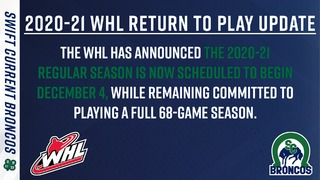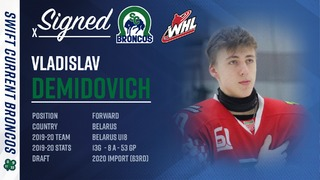Broncos sign Vladislav Demidovich to SPA
6 days ago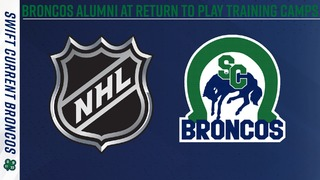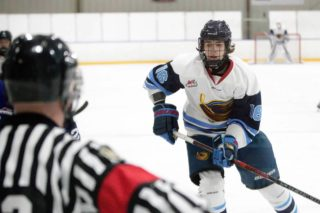Prospect Profile: Kalen Reynolds
1 month ago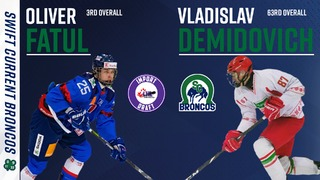14:51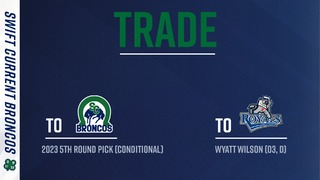Broncos acquire draft pick from Victoria
1 month ago